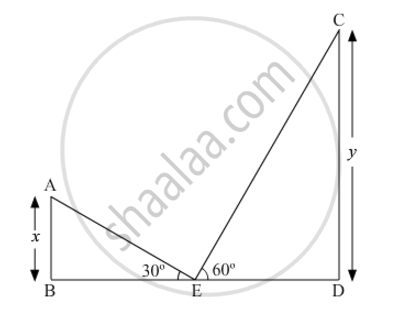# The tops of two towers of height x and y, standing on level ground, subtend angles of 30° and 60° respectively at the centre of the line joining their feet, then find x, y - Mathematics

The tops of two towers of height x and y, standing on level ground, subtend angles of 30° and 60° respectively at the centre of the line joining their feet, then find x, y.

#### Solution

Let AB and CD be the two towers of heights x and y, respectively.Suppose E is the centre of the line joining the feet of the two towers i.e. BD.

Now, in ∆ABE,

(AB)/(BE)=tan30^@

=>x/(BE)=1/sqrt3

=>BE=sqrt3x"  ....(1)"

Also

In ∆CDE,

(CD)/(DE)=tan60^@

=>y/(DE)=sqrt3

=>DE=y/sqrt3"....(2)"

Now, BE = DE   .....(3)       (E is mid-point of BD.)

So, from (1), (2) and (3), we get

sqrt3x= y/sqrt3

=>x/y=1/3

Hence, the ratio of x and y is 1 : 3.

Concept: Heights and Distances
Is there an error in this question or solution?